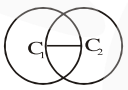# Choose the incorrect statement

Question:

Choose the incorrect statement about the two circles whose equations are given below :

$x^{2}+y^{2}-10 x-10 y+41=0$ and

$x^{2}+y^{2}-16 x-10 y+80=0$

1. Distance between two centres is the average of radii of both the circles.

2. Both circles' centres lie inside region of one another.

3. Both circles pass through the centre of each other.

4. Circles have two intersection points.

Correct Option: , 2

Solution:

$\mathrm{r}_{1}=3, \mathrm{c}_{1}(5,5)$

$\mathrm{r}_{2}=3, \mathrm{c}_{2}(8,5)$

$\mathrm{C}_{1} \mathrm{C}_{2}=3, \mathrm{r}_{1}=3, \mathrm{r}_{2}=3$